# NCERT Area Related to Circles Class 10 Chapter 12 Exercise – 12.1 Solution

Important Points:

Perimeter of or Circumference of Circle = 2πr

Area of Circle = π r²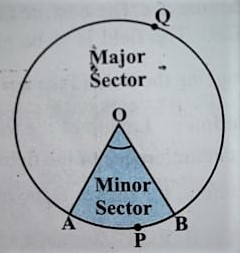Area of Minor Sector = (θ/360º)  πr²

Area of Major Sector = (360º-θ)πr²/360º

Length of Arc = (θ/360º) 2 r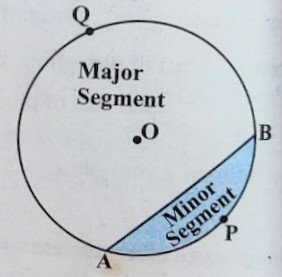Area of Minor Segment = [ (θ/360º) π – sin(θ/2)cos(θ/2)] r²

Area of Major Segment = Area of Circle – Area of Minor Segment

## Exercise – 12.1

Q.1. The Radii of circles are 19cm and 9cm respectively. Find the radius of the circle which has Circumference equal to the sum of the circumferences of the two circles.

Given: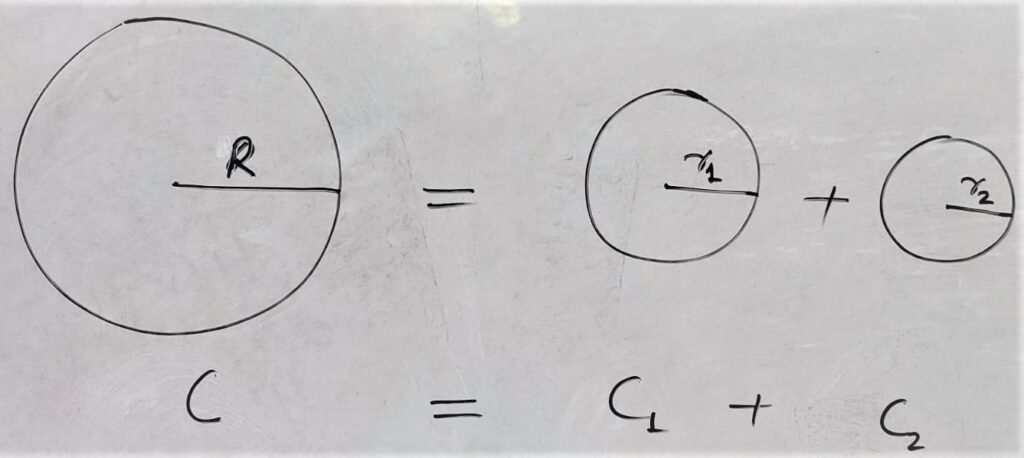r1 = 19cm

r2 = 9cm

To Find:

Radius of New Circle = R =?

Solution:

Circumference of New Circle = Circumference of 1st Circle +Circumference of 2nd Circle

⇒ C                                               = C 1   + C 2

⇒ 2 π R                                          = 2 π r1    + 2 π r2

⇒ 2 π rR                                         = 2 π ( r1 +  r2 )

⇒  R                                                = ( r1 +  r2 )

⇒  R                                                = 19 + 9

⇒ R                                                 = 28 cm

Hence

The radius of the circle which has circumference equal to the sum of the circumferences of the two circles =28 cm

Q.2. The radii of two circles are 8 cm and 6 cm respectively. Find the radius of the circle having area equal to the sum of the areas of the two circles.

Given:

Radius of  1st circle r1 = 8 cm

Radius of  2st circle r2 = 6 cm

To Find:

Radius of New circle =r =?

Solution: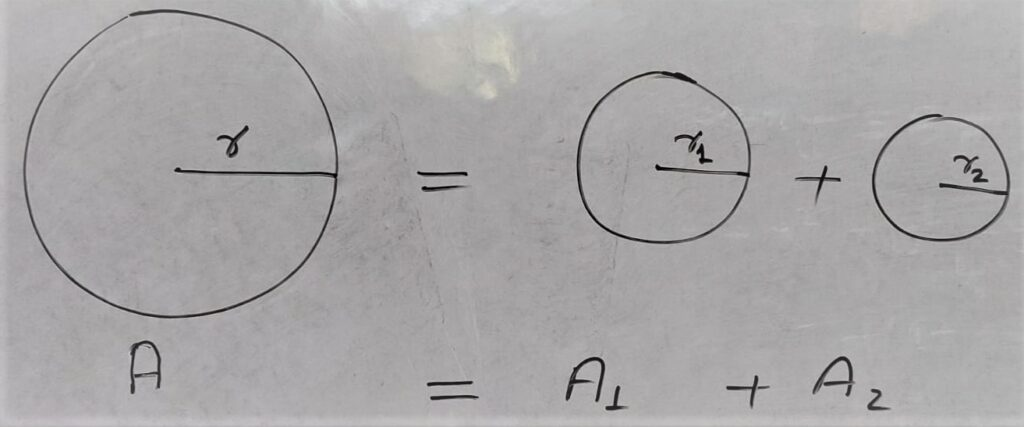Area of New Circle = Area of 1st circle r+ Area of 2st circle r2

⇒ A                                 = A1                                  +  A2

⇒ π r2                            =  π r12                     + π r22

⇒ π r2                            = π (r12                     +  r22)

⇒ r2                                = r12                          +  r22

⇒ r2                                = 82                           +  62

⇒ r2                                = 64                           + 36

⇒ r2                                = 100

⇒ r                               = √100

⇒ r                                 = 10

Hence Radius New Circle = 10 cm.

Q.3. Figure 12.3 depicts an archery target marked with its five scoring regions From the center outwards as Gold , Red , Blue , Black and White . The Diameter of the region representing Gold score is 21 cm and each of the other bands is 10.5 cm wide. Find the area of each of the five scoring regions.

Given: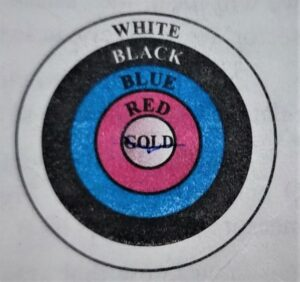Total Scoring regions = 5

Diameter of Gold Region = 21 cm

Width of Each band = 10.5 cm

To Find:

Radius of Gold Region r = 21/2 = 10.5 cm

Area of Gold Region = π r²

⇒ Area of Gold Region = 22/7 × 21/2 × 21/2

⇒ Area of Gold Region =( 11 × 3 × 21 )/2

⇒ Area of Gold Region = 693/2

⇒ Area of Gold Region = 346.5 cm²

Radius of red region = 10.5 + 10.5 = r + r = 2 r

Area of Red Region = Area of Circle with Radius 2r – Area of Gold Region

⇒  Area of Red Region = π (2r)² – π (r)²

⇒  Area of Red Region = π 4r² – π r²

⇒  Area of Red Region = 4 πr² – πr²

⇒  Area of Red Region = 3 πr²

⇒  Area of Red Region = 3 × 346.5

⇒  Area of Red Region = 1039.5 cm²

Similarly

Area of Blue Region = π (3r)² – π (2r)²

⇒ Area of Blue Region = π 9r² – π 4r²

⇒ Area of Blue Region = 9 πr² – 4 πr²

⇒ Area of Blue Region = 5 πr²

⇒ Area of Blue Region = 5 × 346.5

⇒ Area of Blue Region = 1732.5 cm²

Area of Black Region = π (4r)² – π (3r)²

⇒ Area of Black Region = π 16r² – π 9r²

⇒ Area of Black Region = 16πr²  – 9πr²

⇒ Area of Black Region = 7πr²

⇒ Area of Black Region = 7 × 346.5

⇒ Area of Black Region = 2425.5 cm²

Area of White Region = π (5r)² – π (4r)²

⇒ Area of White Region =π 25r² – π 16r²

⇒ Area of White Region = 25 πr² – 16 πr²

⇒ Area of White Region = 9 πr²

⇒ Area of White Region = 9 × 346.5

⇒ Area of White Region = 3118.5 cm²

Q.4. The Wheels of a car are of diameter 80 cm each. How may complete revolutions does each wheel make in 10 minutes. When the Car is travelling at a speed of 66 km per hour ?

Given:

Diameter of Each Wheels of Car = 80 cm

Speed of Car = 66 km/hr

To Find:

Number of complete revolutions in 10 minutes = ?

Solution:

Speed of car = 66 km/hr

In 1 hour = 60 minutes distance covered by car = 66 km

In 1 minute distance covered by car =66/60 = 11/10 = 1.1 km

In 10 minutes distance covered by car = 1.1×10 =11 km = 11×1000×100 cm { 1 km = 1000 m; 1 m = 100 cm }

Radius of each wheel = 80/2 = 40 cm.

One Revolution = Circumference of each wheel = 2 πr

⇒ One Revolution = 2 × 22/7 × 40 cm =

Total Distance Covered by in 10 minutes = 11 × 1000 × 100 cm

Number of Revolution by Each wheel = Total distance in 10 minutes /1 Revolution

⇒ Number of Revolution by Each wheel = ( 11 × 1000 × 100 )÷ 2 × 22/7 × 40

⇒ Number of Revolution by Each wheel = ( 1100000 × 7 ) ÷ (44 × 40)

⇒ Number of Revolution by Each wheel = 7700000 ÷ 1760

⇒ Number of Revolution by Each wheel = 4375

Hence Total Number of Revolution by Each wheel = 4375

Q.5. Tick the correct answer in the Following and justify your choice: If the perimeter and the area of a circle are numerically equally equal , then the radius of the circle is:

(A) 2 units

(B) π units

(C) 4 units

(D) 7 units

Explanation:

Area of circle = Perimeter of circle

⇒ π r2 = 2 πr

⇒ π r2 = 2 πr

⇒ r2 = 2 r

⇒ r2 = 2 r

⇒ r2 /r= 2

⇒ r = 2 units